Maths-
General
Easy

Question

# In what time will Rs1500 yield  Rs1996.50 as compound interest at 10% per annum compounded annually?Hint:

## The correct answer is: 3 years

### Complete step by step solution:We calculate the total amount by the formula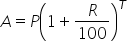…(i)where P is Principal amount, T is number of years and R is rate of interestHere, we have R = 10% ,P = 1500 , A = 1996.5 and T = ?On substituting the known values in (i), we get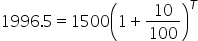On dividing both the sides by 1500, we have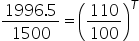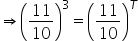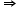T = 3 yearsIn 3 years will Rs 1500 yield Rs 1996.50 as compound interest at 10% per annum compounded annually.#### With Turito Foundation.#### Get an Expert Advice From Turito.